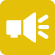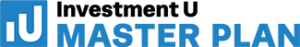## 1-Minute Survey

What Type of Investor Are You? – Take This 1-Min Survey to Find Out

Financial Literacy

# What is Present Value (PV)?

Value is a fluctuating concept that’s ever-changing. One of the core principles of accounting states that money received in the future isn’t worth as much as an equal sum received today. This is why present value (PV) is such an important concept. It’s the current value of a future sum of money. It represents the value of that sum at the current point in time vs. its expected value in the future.

Economists, investors, accountants, and financial professionals use present value as a benchmark for understanding forward-looking values. These values are important for contextualizing everything from inflationary losses to real rate of return.## Present Value vs. Future Value

The best way to illustrate present value vs. future value is through example. Consider the value of \$1,000 today vs. its value five years from now in the following situation.

If you invest \$1,000 today at a 5% annualized return, its value will be \$1,276.28 in five years. Compare this to the \$1,000 you receive outright in five years. Today’s \$1,000 is worth \$276.28 more at the same time. It works in reverse, too. If you apply a discount rate of 5% to \$1,000 five years from now, it’ll be worth \$783.53 today.

There are also inflationary considerations that make present value worth more than future value. A computer that costs \$1,000 at today’s prices may face 2% inflation over five years and cost \$1,150 in the future. The value of your \$1,000 in the present day is higher because it offers greater buying power.

Interest rates and the rate of inflation have the power to change the value of a sum over time. They’re the difference between losing money by doing nothing and maximizing present value vs. implied future devaluation.

## How to Calculate Present Value

Situations involving present value tend to involve future value. You’re calculating PV to determine if a proposed future value has equivalent (or better) value in the present day. To calculate PV, investors need to know the future value, number of periods and the rate of return for an investment. Together, these variables factor into the following equation:

PV = FV (1/(1+rn))

• PV = present value
• FV = future value
• r = rate of return
• n = number of periods

Again, an example is the best explanation. Given the choice between \$1,000 invested at 5% for one year and \$1,100 outright a year from now, which option is better? To make that determination, you’d calculate the present value. What you’d find is that the PV of \$1,100 received one year from now is actually \$1,047.60, which is higher than the \$1,000 you could have outright.

## Why is Present Value Important?

Present value is widely used by long-term investors as a way to gauge the value of an investment based on its rate of return. For example, real estate investors will use PV to calculate their future value stream from rent payments. Another example of PV is its use when businesses consider acquiring an asset or another business. They’ll calculate present value using future earnings projections.

Above all, present value is a useful tool in vetting investments. The promise of a ballpark return doesn’t automatically make that investment a sound one. It’s important to calculate PV as a way of determining real value—then comparing those values across investment opportunities.

## Present Value vs. Net Present Value

There’s a subtle difference between these two terms. Where PV focuses at determining the value of a future sum or income stream in today’s dollars, net present value (NPV) also takes into account the presence of cash outflows during that period. For example, net present value accounts for the initial capital investment.

NPV is a steadfast tool in capital project budgeting for companies, as well as real estate investments. Assessing NPV can shed light into how long the project will take to clear the investment hurdle to profitability, as well as what the annualized ROI looks like. Positive NPV means the discounted value of all future cash flows will also be positive.

## The Drawbacks

PV is far from a perfect metric and can involve a considerable amount of educated guessing. For starters, it can be difficult to nail down an accurate discount rate due to fluctuating interest rates. There’s also guesswork involved when it comes to cost of capital, which can skew the NPV.

PV and NPV also suffer from uncontrollable external factors. Inflation might ebb and flow year to year over the course of an investment. An investor might need to pull out early. Rates of return might change due to the nature of the asset. Every change surrounding the parameters of the future value changes the nature of its present value. It can be nearly impossible to account for them all—especially in volatile environments or within specific investments.

## A Dollar Today is Worth More Than a Dollar Tomorrow

This old adage applies when it comes to thinking about the present value of currency vs. its implied future value. Money today is money that you can invest and use to generate ROI. Money of the same sum received next year is already the victim of inflation

If someone offers to give you a sum of money today vs. an amount next year, the right answer is to compare present values. If the present value of the future investment is higher, bide your time. If the present value of today’s sum is higher, park that money to earn an interest rate above and beyond the rate of inflation for the amount of time you plan to invest it.